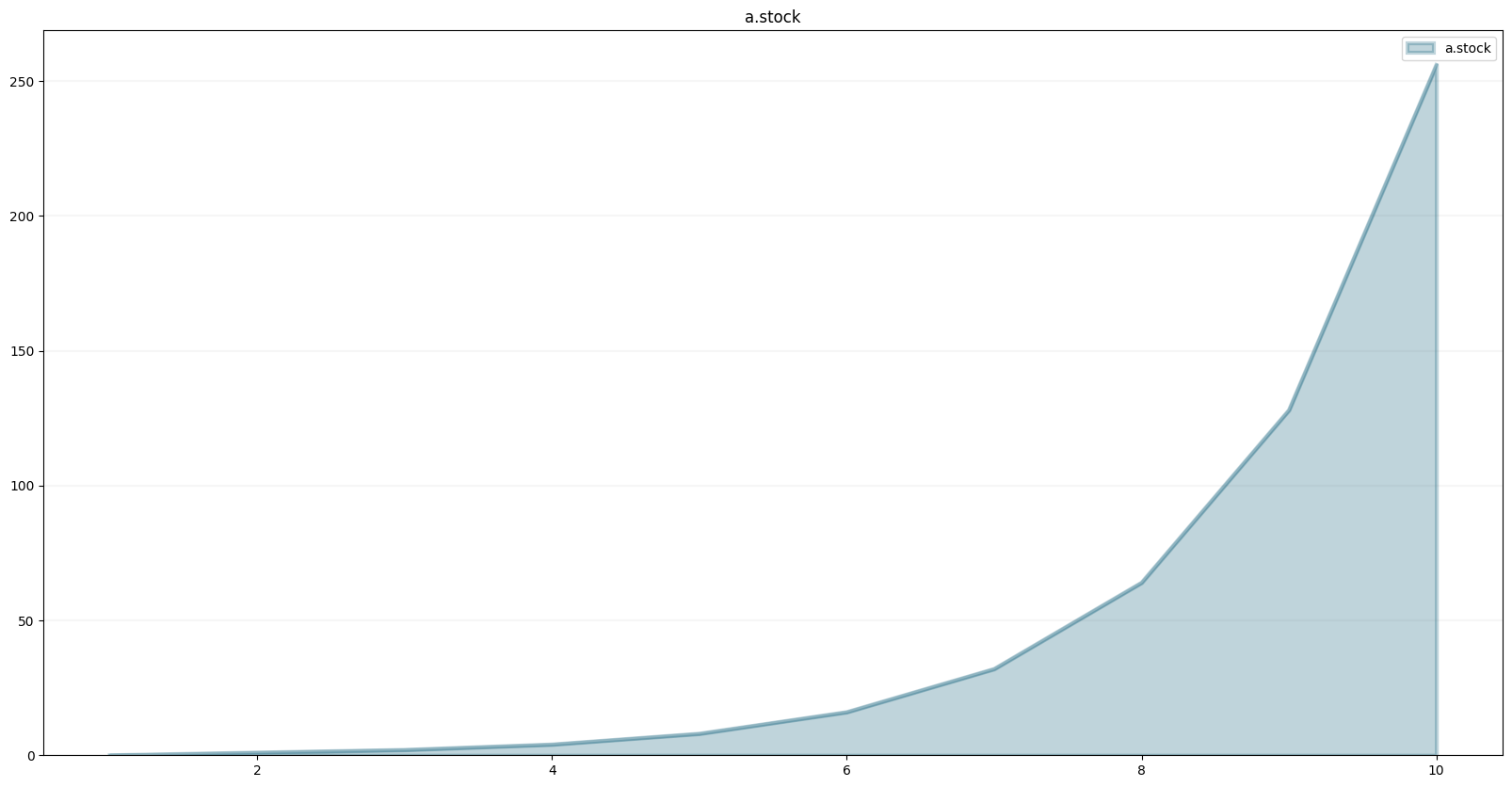BPTK API Documentation for the Module class

# Module

## Module Constructor

Module(model, name, parent=None)

The Module class is used to structure SD DSL models into individual modules.

Modules can be nested.

If you create model elements such as stocks, flows and converters via the module, the elments are added to the model, but the element names are turned into fully qualified names of the form parent_module_name.module_name.name.

The fully qualfied name is used as the equation name in the Model class and is needed when making calls to bptk.run_scenario or bptk.plot_scenario.

Check the Beergame or Enterprise Digital Twin models to see the module class in action.

## Module.biflow

biflow(name)

Add a Biflow to the underlying model. The name of the biflow will be a fully qualified name consisting of all nested module names plus the actual element name using dot notation, i.e. namespace.name

## Module.constant

constant(name)

Add a Constant to the model. The name of the constant will be a fully qualified name consisting of all nested module names plus the actual element name using dot notation, i.e. namespace.name

## Module.converter

converter(name)

Add a Converter to the model. The name of the converter will be a fully qualified name consisting of all nested module names plus the actual element name using dot notation, i.e. namespace.name

## Module.flow

flow(name)

Add a Flow to the model. The name of the flow will be a fully qualified name consisting of all nested module names plus the actual element name using dot notation, i.e. namespace.name

## Module.fqn

fqn(name)

Given a name this returns the fully qualified name, i.e. name prefixed by the module namespace.

The namespace is defined by the names of all parent modules, e.g. parent_module_name.module_name

• Parameters

name – String The name that is to be converted into a fully qualified name.

• Return

Return the fully qualified name, i.e. namespace.name

## Module.initialize

initialize(module,…)

Override this method in concrete Module subclasses and use it to define the model using Stocks, Flows, Converters and Constants.

Pass in any module dependencies as parameters.

• Parameters

** module ** Module subclass External module that contains model elements that are needed within the current module

## Module.stock

stock(name)

Add a Stock to the model. The name of the stock will be a fully qualified name consisting of all nested module names plus the actual element name using dot notation, i.e. namespace.name

# Usage

Let’s create a simple example model containing to modules A and B that depend on each other.

``from BPTK_Py import Model, Module``

Set up a model that runs over 10 timesteps:

``model = Model(starttime=1,stoptime=10,dt=1,name='model')``

Now create to module classes that define the actual model:

``````class A(Module):

def initialize(self,b):
## stocks

stock = self.stock("stock")

## flows.

flow = self.flow("flow")

## equations

stock.initial_value = 0.0
stock.equation = flow

flow.equation = b.stock("stock")``````
``````class B(Module):

def initialize(self,a):

## stocks
stock = self.stock("stock")

## flows.
flow = self.flow("flow")

## equations
stock.initial_value = 1.0
stock.equation = flow

flow.equation = a.stock("stock")``````

Initialize the modules:

``````a = A(model,"a")
b = B(model,"b")

a.initialize(b)
b.initialize(a)``````

Plot the graph for the a_module.a_stock element:

``model.stock("a.stock").plot()``Of course you can also access the model element directly via their respective modules:

``a.stock("stock").plot()``In practice you will probably set up and scenario managers and then access the model elements via the bptk class.

``````from BPTK_Py import bptk
bptk = bptk()``````
``````bptk.register_scenario_manager(
{
"sm":
{
"model":model,
"scenarios":
{
"base":{}
}

}

}
)``````
``````bptk.plot_scenarios(
scenario_managers=["sm"],
scenarios=["base"],
equations=["a.stock","b.stock"]
)``````During model development, it can be useful to list all model equations:

``bptk.list_equations()``
``````Available Equations:

Scenario Manager: sm
Scenario: base
--
stock:          a.stock
stock:          b.stock
flow:           a.flow
flow:           b.flow
``````